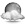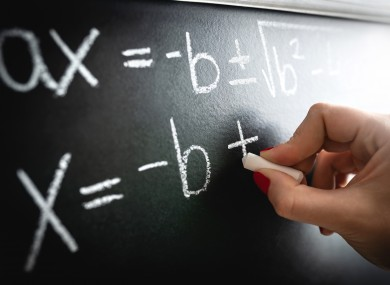This site uses cookies to improve your experience and to provide services and advertising. By continuing to browse, you agree to the use of cookies described in our Cookies Policy. You may change your settings at any time but this may impact on the functionality of the site. To learn more see our Cookies Policy.
OK
Dublin: 3 °CMonday 11 November, 2019

# Maths Week: The solutions to all of the puzzles

## Here are all the answers to the Maths Week puzzles posted yesterday.How did you get on with yesterday's puzzles?
Image: Shutterstock/Tero Vesalainen

MATHS WEEK BEGAN yesterday and to celebrate, we posted 10 puzzles last night that may have left you scratching your head.

If this was the case, here are the answers you’ve been waiting for.

Let us know how you did in the comments.

Question 1

Which is the smallest value?

a) 67%
b) 2/3
c) 8/13
d) 0.666

We can compare them if we convert all to decimals.
a) 67% is 0.67, b) 2/3 is 0.666 (approximately) c) 8/13 is 0.615 and d) is 0.666

Question 2

What is one half of two-fifths of 20 times 3?

a) 12
b) 25
c) 5
d) 14

20 times 3 is 60
One fifth of 60 is 12
Two fifths of 60 therefore is 24
One half of 24 is 12.

Question 3

A recent TheJournal.ie report on energy drinks told us that over 26 million litres of energy drinks are consumed in Ireland per year. One product popular with young people is sold in 500ml cans. Each of these contains 55g of sugar. Suppose someone was to drink 1 can of this a day for a year, how much sugar would they consume in a year from this source alone?

a) 2.17 kg
b) 3.65 kg
c) 20.075 kg
d) 5.5 kg

55g a day for 365 days would be 55 x 365 = 20,075 g. There are 1000 grams in 1 kilogram, so that makes 20.075kg.

Question 4

Last Thursday was the 10th day of the 10th month. 10 multiplied by 10 is 100, which of course is a square number. How many more times will this happen (ie. the product of the day and the month being a square number) before the end of this year?

a) 1
b) 2
c) 3
d) 4

The dates are 11/11, 3/12 (3 x 12 = 36 which is the square of 6), 12/12, 27/12 (27 x 12 = 324 which is the square of 18).

Question 5

The following shape, equivalent to five squares, was cut out of cardboard to make a box. The perimeter is 72 cm. What is the area of cardboard in centimetres squared?a) 72 cm sq
b) 120 cm sq
c) 180 cm sq
d) 144 cm sq

The perimeter is 72 units. There are 12 equal edges on the perimeter.
Therefore each edge is 72/12 = 6 units long.
Each square therefore has an area of 6×6 = 36
There are 5 of these squares, so total area is 5 x 36= 180

Question 6

Which is the best value?

a) Half a square metre for €10
b) Half a metre squared for €5
c) They are both the same
d) None of the above

Answer: c) They are both the same

Half a square metre for €10 is equivalent to a square metre for €20.
Half a metre squared is 0.5m x 0.5m = 0.25m2 which is a quarter of a square metre which for €5 is also equivalent to €20 per square metre.

Question 7

My age is 4 times what it was 4 years ago minus twice what it will be in 4 years’ time. What’s my age?

a) 16
b) 18
c) 24
d) 26

If we let Y represent my age, then we can convert the word statement into a mathematical statement as follows.

Y = 4 (Y-4) – 2(Y + 4)
Y = 4Y – 16 – (2Y + 8)
Y = 4Y – 16 – 2Y -8
Y = 2Y -24

Subtracting 2Y from each side we get
-Y = -2

Multiplying both sides by -1 we get
Y = 24.

Question 8

Which is best value per litre from these special offers of the same product originally marked €4 per litre?

a) 1 litre for €3.49
b) 1 litre with 20% off marked price
c) 3 for the price of 2
d) 33% extra free

Answer 8: c) 3 for the price of 2

a) Is €3.49 a litre.
b) 20% off marked price is 20% off €4. 20% of €4 is €0.80. So the price is €3.20 per litre.
c) 3 for the price of 2 is 3 litres for €8. Which is 8/3 = €2.67 per litre.
d) 33% extra free is 1.33 litres for €4. This would be 4/1.33 = €3 per litre.

Question 9

The Government increased the carbon tax in the budget by €6 per tonne of carbon dioxide (CO2) released. A petrol car can emit 2.31kg of carbon dioxide from burning 1 litre of fuel. How much extra would this cost for a petrol car with a fuel efficiency of 16 km/l driving 10,000 km in a year?

a) €56
b) More than €100
c) €28
d) Less than €10

This car, driving 10,000 km at 16 km/l will use 10,000/16  = 625 litres of petrol.
Burning one litre of petrol will emit 2.31 kg of CO2. 625 litres will cause 626×2.31 = 1443.75 kg emissions. That is 1.443 tonnes
If the carbon tax charges and extra €6 per tonne then the extra cost will be
€6×1.443 = €8.66

Question 10

The following maths puzzle went viral in Japan, as most people will fall into the same trap and get the incorrect answer. Can you solve it correctly?

9 – 3 ÷ ⅓ + 1 = ?

Order of operations is important here. BIMDAS is an acronym that helps us remember the order in which operations should be done so that everyone gets the same answer.

It tells us Brackets – Indices (powers) – Multiplication – Division – Addition – Subtraction. In the above statement the division must be done first:

-3  ÷ ⅓ = -9

So => 9 – 9 + 1

= 0 + 1

= 1